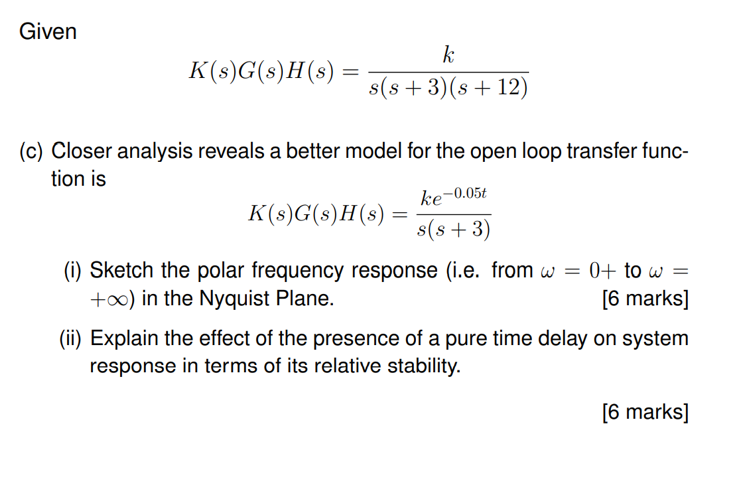Home / Expert Answers / Mechanical Engineering / given-k-s-g-s-h-s-frac-k-s-s-3-s-12-c-closer-analysis-reveals-pa150

# (Solved): Given $K(s) G(s) H(s)=\frac{k}{s(s+3)(s+12)}$ (c) Closer analysis reveals ...???????

Given $K(s) G(s) H(s)=\frac{k}{s(s+3)(s+12)}$ (c) Closer analysis reveals a better model for the open loop transfer function is $K(s) G(s) H(s)=\frac{k e^{-0.05 t}}{s(s+3)}$ (i) Sketch the polar frequency response (i.e. from $$\omega=0+$$ to $$\omega=$$ $$+\infty)$$ in the Nyquist Plane. [6 marks] (ii) Explain the effect of the presence of a pure time delay on system response in terms of its relative stability.

We have an Answer from Expert